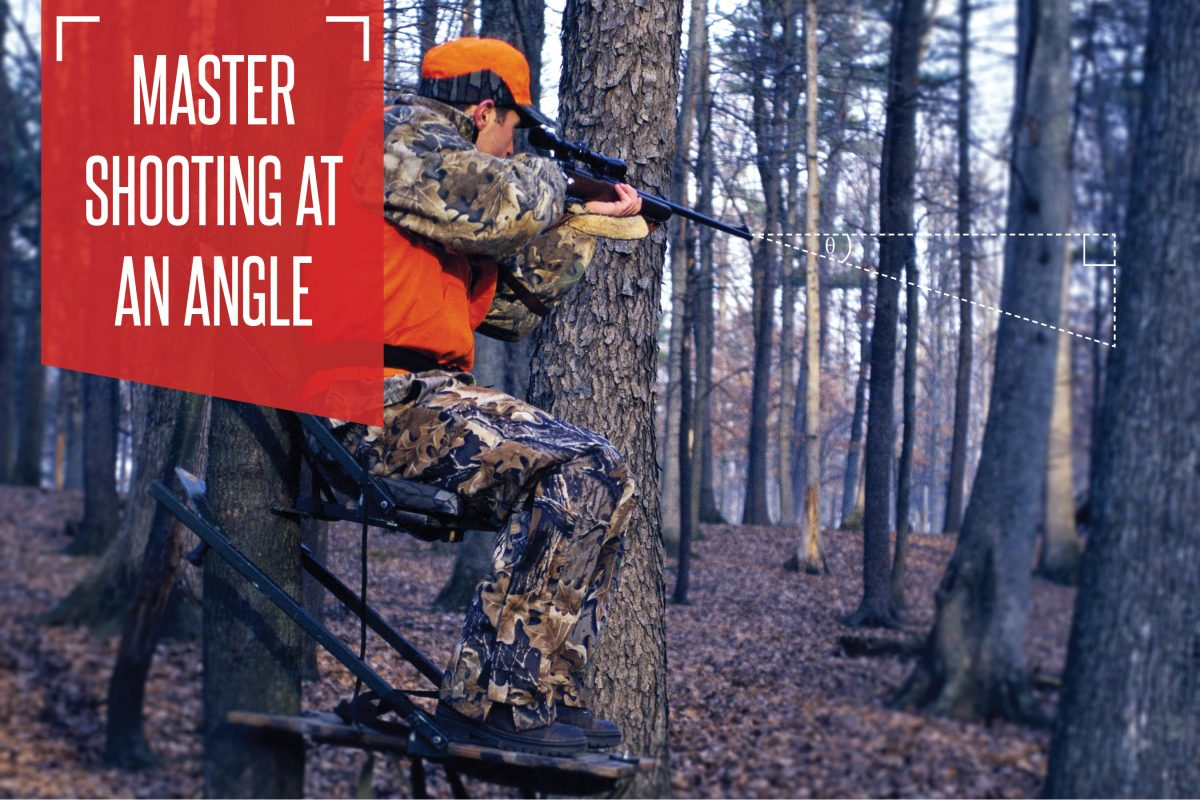# Master shooting at an angle

Despite all the confusion surrounding where to aim when shooting at a steep angle, once you know the principles involved, hitting the mark is really quite simple.

“Shoot high.” “Aim low.” “It doesn’t matter, just aim for the middle.” If you went to a typical hunting camp and took a poll on where to aim on a steep angle shot, you would probably get almost as many answers as hunters, and lots of “it depends.” The correct answer is aim low (I’ll explain why below). But how low?

Before we can calculate how low to aim, we first need to understand the principles involved. Due to the force of gravity acting on the bullet, the farther away a target is, the more a bullet drops. Conversely, the closer a target is, the less the bullet drops. So, if you are aiming at a target 200 yards away and then switch to a target 100 yards away, you will aim lower in relation to the target. Pretty simple so far.

Here’s where it starts to get a little complicated. Gravity acts on a bullet in a downward direction. This means that it only deflects a bullet’s path away from horizontal. Consider this: If you were to drop a ball at a target 10 feet below you, you would simply hold the ball directly above the target and let it fall straight down. But if the target was 10 feet in front of you, you would need to aim higher than the target in order to hit it.

Think of yourself in relation to the target using a right triangle. The horizontal distance is one side, your height above or below the target is the other side, and the line of sight to your target is the hypotenuse. Remembering our basic geometry, the hypotenuse is longer than either side, meaning the line of sight distance between you and your target is always going to be greater than the horizontal distance.

Next, estimate the angle you are shooting below (or above) horizontal. You can do this using a mildot-master or even a compass with an inclinometer. Thanks to trigonometry, we know that if we multiply the line-of-sight distance by the cosine of the angle we just estimated, we will get the horizontal distance. For this step, it may be handy to keep a notecard with cosine values in your pack. Below is a table of cosine values.